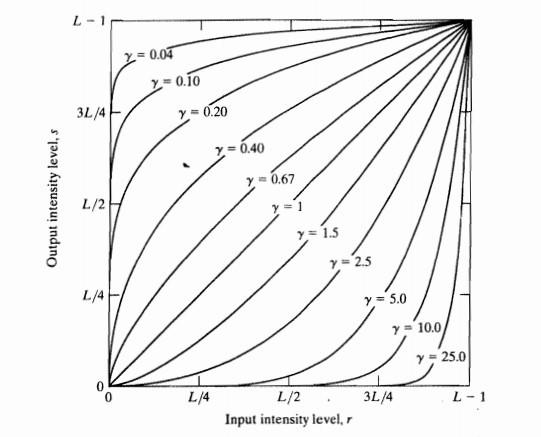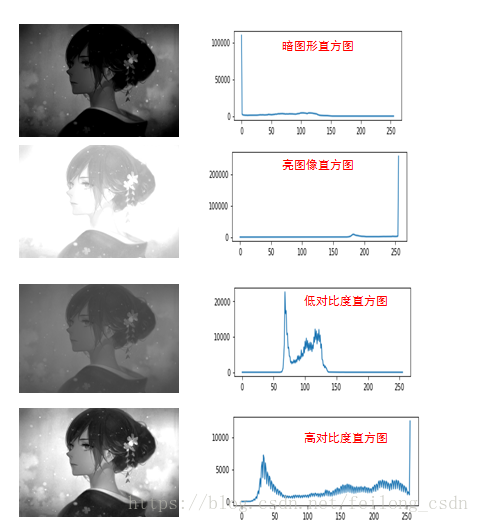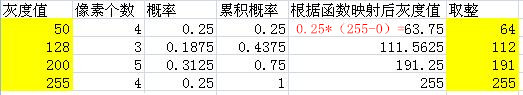学习OpenCV-Python——图像增强

图像增强

• 领域处理技术。对像素点及其周围的点进行处理，即使用卷积核。
• 点处理技术。只对单个像素进行处理。

归一化

cv2.normalize(src, dst, alpha, beta, norm_type, dtype, mask) → dst

src： 原图像对象

dst： 经过转化后的图像对象

alpha： 归一化后灰度像素最小值，一般为0

beta： 归一化后灰度像素最大值，一般为255

norm_type： 归一化的类型，可以取以下值

(1) cv2.NORM_MINMAX： 数组的数值缩放到一个指定的范围，线性归一化，一般较常用
(2) cv2.NORM_INF:归一化数组的C-范数(绝对值的最大值)
(3) cv2.NORM_L1 : 归一化数组的L1-范数(绝对值的和)
(4) cv2.NORM_L2: 归一化数组的(欧几里德)L2-范数

类型转换

dst = cv2.convertScaleAbs(src)

点处理技术

线性变换

$y = a*x+b$

a>1： 增强图像的对比度，图像看起来更加清晰
a<1： 减小了图像的对比度， 图像看起来变模糊
a<0 and b=0：图像的亮区域变暗，暗区域变亮
a=1 and b≠0：图像整体的灰度值上移或者下移，也就是图像整体变亮或者变暗，不会改变图像的对比度，b>0时图像变亮，b<0时图像变暗
a=-1 and b=255：图像翻转

分段线性变换

$\begin{cases} y = a_1*x+b & x<x_1 \\ y = a_2*x+b & x_1<x<x_2 \\ y = a_1*x+b & x_2<x \end{cases}$

对数变换

$y =c* log(1+x)$

幂律变换/gamma变换

$y = c*x^γ$

γ > 1： 处理漂白的图片，进行灰度级压缩

γ < 1： 处理过黑的图片，对比度增强，使得细节看的更加清楚图像直方图均衡化图像滤波

1.平滑滤波

2.锐化滤波

平滑滤波

#均值滤波
blur_img = cv2.blur(img, 5)
#中值滤波
blur_img = cv2.medianBlur(img, 5)
#高斯滤波
blur_img = cv2.gaussianBlur(img,5)